# Electronics and Communication Engineering - Automatic Control Systems

Exercise : Automatic Control Systems - Section 1
11.
For the given figure C(s)/R(s)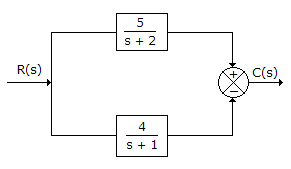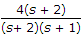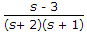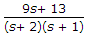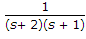Explanation: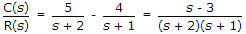.

12.
A stepper motor is
a two phase induction motor
is a kind of rotating amplifier
is an electromagnetic transducer used to convert an angular position of shaft into electrical signal
is an electromechanical device which actuates a train of step angular movements in response to a train of input pulses on one to one basis
Explanation:

When input pulses are applied, the motor moves in steps.

13.
In a two phase ac servomotor rotor resistance is R and rotor reactance is X. The speed curve will be linear if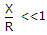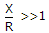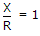X2 = R
Explanation:

In a two phase servomotor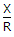∠ < 1 so that torque speed curve is almost linear.

14.
In the given figure, the input frequency is such that R = XC. Then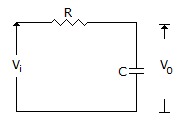v0 lags vi by 45°
v0 lags vi by 90°
Explanation:

R = XC, voltage drops across R and XC are equal and at 90° with respect by each other. V0 lags Vi by 45°.

15.
From the noise point of view, bandwidth should
be large
not be too large
should be as large as possible
should be infinite